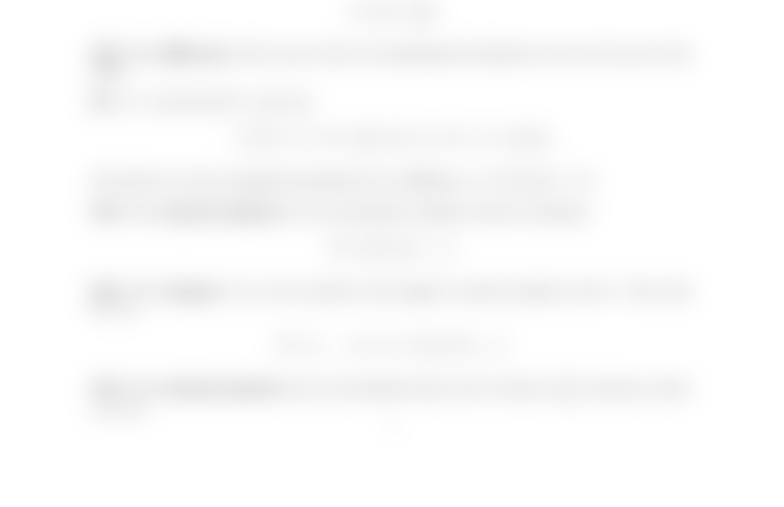Study Guides (400,000)
US (230,000)
NCSU (600)
MA (50)
MA 141 (40)
Final

# MA 141 Lecture 1: Real and Complex Number SystemsExam

Department
Mathematics
Course Code
MA 141
Professor
Kevin Flores
Study Guide
Final

This preview shows half of the first page. to view the full 2 pages of the document.MA 141 Chapter 0
Section 0.1: Real and Complex Number Systems
Def: Aset is a collection of objects. If xis an object in a set S, we say xis an element of
Sand we write xS.
If every element of a set Ais also an element of a set B, we say Ais a subset of Band
write AB.
Two sets are equal if they have exactly the same elements. Then we have
AB, B AA=B.
Def: The union of two sets is the set containing all elements of either set.
Ex: A={1,2}and B={2,3,4}.
AB={1,2,3,4}
Def: The intersection of two sets is the set containing the elements that are in both sets.
Ex: A={1,2}and B={2,3,4}.
AB={2}
Def: The diﬀerence of two sets is the set containing the elements in one set but not in the
other.
Ex: A={1,2}and B={2,3,4}.
A\B=AB={1}, B \A=BA={3,4}
Note there are two acceptable notations for set diﬀerence, A\Band AB.
Def: The natural numbers,N, are all positive numbers with no decimals.
N={1,2,3,4, . . .}
Def: The integers,Z, are the positive and negative natural numbers and 0. Note that
NZ.
Z={. . . , 3,2,1,0,1,2,3, . . .}
Def: The rational numbers,Q, are all numbers that can be written as a
b, a fraction, where
a, b Z.
1